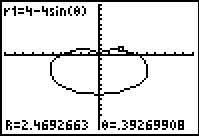Polar Graphing
To graph an equation in polar form, set the calculator MODE to polar (POL).→
 If you want to see your TRACE in terms of r and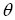, change FORMAT (2nd ZOOM) to PolarGC.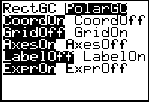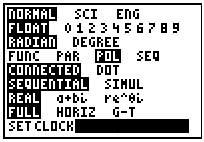Examples:
1.  Graph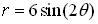Enter the equation into Y=: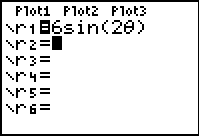Choose ZOOM #6 ZStandard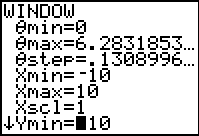Choosing #6 ZStandard will set the window as follows:with the x and y values in the normal -10 to10 configuration

Graph
(using the ZOOM #6 ZStandard)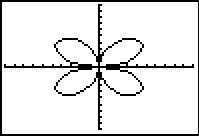Because the  Standard viewing window has a 3 to 2 aspect ratio (it is not evenly divided on both axes)., the graphs may appear slightly distorted.

Graph
(using the ZOOM #5 ZSquare)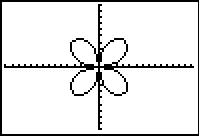To prevent distortion,
choose ZOOM #5 ZSquare.

 Choosing #5 ZSquare will also set the window as follows:but with the x and y values in the -15.16129 to 15.16129 configuration.

Trace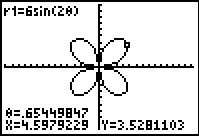Notice that x and y are displayed.  The calculator FORMAT is set to the default value of RectGC.

TraceNotice that r andare displayed.  The calculator FORMAT is set to PolarGC.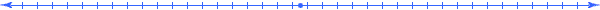2.  Graph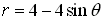Enter the equation into Y=: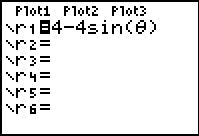Choose ZOOM #5 ZSquare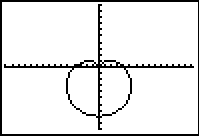Trace3.  Find r from the graph screen ofwhen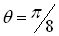You can find the value of a function from the graph screen in two ways:

 Method 1: -- by using the VALUE function      in the CALC (2nd TRACE) menu:This screen will appear....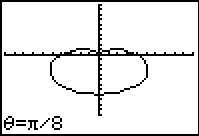If the FORMAT (2nd ZOOM) is set to RECTGC you will see the x and y coordinates...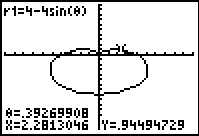If the FORMAT is set to POLARGC you will see the r and theta coordinates...Method 2: -- simply hit TRACE and enter the theta value right on the graphing screen.If the FORMAT is set to POLARGC, you will see the r value answser.